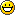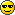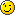## Laboratorul 10 - Prezentul și Viitorul criptării cu chei publice (Public Key Encryption)

Prezentarea PowerPoint pentru acest laborator o puteți găsi aici.

În acest laborator vom face niște exerciții strașnice folosind metode de criptare cu chei publice pentru schimb de chei (key exchange) și criptare de date.

### Exercițiul 1: Diffie-Hellman key exchange (4p)

Așa cum am discutat la curs, Diffie și Hellman au propus primul mecanism de schimb de chei astfel încât două părți care nu partajează niciun secret a priori să poată stabili un secret comun. Acest mecanism oferă posibilitatea ca cele două părți să aibă un secret comun pe care doar ele să îl știe, chiar dacă schimbul de mesaje este vizibil și unor părți terțe (cu excepția unui atac activ de tip man in the middle care poate modifica conținutul schimbului de mesaje, atac care poate fi rezolvat folosind TLS/certificate, dar nu vor face parte din subiectul acestui laborator).

Descărcați codul laboratorului de aici. După dezarhivare, veți găsi codul sursă pentru un client (dhe.c) și pentru un server (dhe_server.c), împreună cu un Makefile și niște parametri fixați p și g în fișierele dhparam.pem.

Clientul și serverul au o structură similară. Fiecare are trebui să construiască o cheie publică, pe care să o trimită celeilalte părți, iar în final să își calculeze cheia secretă. Scopul vostru este să completați părțile lipsă din cod. Pentru aceasta, consultați documentația openssl de aici. Din moment ce sunt similare, concentrați-vă numai pe unul dintre ele și completați în mod asemănător și în cealaltă parte.

Fișierul Makefile ar trebui să vă ajute să faceți build la ambele. Folosiți comanda 'make all'. După completarea TODO-urilor necesare din fișier, puteți porni server-ul folosind comanda 'make start_server' și clientul folosind comanda 'make start_client'.

Dacă totul merge bine, ar trebui să vedeți același secret atât la client, cât și la server.

Înainte să începeți acest task, verificați dacă aveți openSSL instalat (în acest laborator vom folosi openSSL 1.1.1):

#openssl version

De asemenea, asigurați-vă că aveți “libssl-dev” instalat (rugați pe asistenții de laborator să vă ajute dacă lipsesc, de exemplu dacă nu se găsesc fișierele header la compilare).

Dacă aveți nevoie să instalați openSSL pe calculatorul vostru, descărcați openssl 1.1.1 de aici. Salvați fișierul într-un folder local accesibil, apoi compilați-l și instalați-l într-un folder. Deschideți folder-ul în bash și rulați următoarele comenzi:

linux$./config --prefix=/home/student/local --openssldir=/home/student/local/openssl linux$ make
linux$make test linux$ make install

(în caz de probleme, verificați și instrucțiunile de la finalul acestui laborator).

Nu uitați să actualizați fișierul Makefile cu path-urile spre folderele voastre de instalare.

În timp ce se face build / se compilează, puteți începe să lucrați la celelalte exerciții.

### Introduction to Post Quantum Cryptography

Do not be scared by the fancy name! Post Quantum Cryptography has nothing “Quantum” in it (beside the name). In the following lines we will briefly introduce you to this new concept.

#### Present Public Key Cryptography

In modern public key cryptography the security of algorithms relies on hard to solve mathematical problems. The motivation behind it is that if you want to build an encryption scheme you have to prove somehow that the scheme is secure. What better way to satisfy this than building it in such a way that breaking its security relies on solving hard mathematical problems? Humanity has failed collectively to solve them and one would consider these failed attempts as attempts to break the security of the scheme!

The security of popular public key algorithms used today relies on one of these hard mathematical problems: integer factorization problem (RSA), discrete logarithm problem (DH) or elliptic-curve discrete logarithm problem (ECC).

In a breakthrough paper (https://arxiv.org/pdf/quant-ph/9508027.pdf), Peter Shor an American mathematician invented in 1994 an algorithm that can perform integer factorization in polynomial-time. Additionally it can also be used for computing the discrete logarithm problem. In simple words, this algorithm breaks public key algorithms used in the present! The catch (and this is why life is still beautiful and we can safely secure our data) is that this algorithm, in order to be efficient, needs to run on an efficient enough Quantum Computer. But are Quantum Computers feasible in practice? If so, what can we do?

Shor's algorithm is a quantum algorithm that can solve the factorization problem in polynomial time and additionally can be used to compute the discrete logarithm problem breaking the public key algorithms used nowadays. From the threat of an efficient enough Quantum Computer running the Shor's algorithm emerges the motivation and interest for Post Quantum (Quantum-proof) Cryptography!

While Quantum Algorithms are not the object of this course and this algorithm is considered one of the most complex Quantum Algorithms, you can find here (https://www.scottaaronson.com/blog/?p=208) a “gentle” introduction to Shor's algorithm!

For a full summary of the impact of Quantum Computers on present Cryptography we recommend this nice article: https://arxiv.org/pdf/1804.00200.pdf!

#### Post Quantum Cryptography

Having this in mind we can finally define what is a “Post Quantum Algorithm”:

A Post Quantum Algorithm is an algorithm that is considered secure even if an adversary has a powerful enough Quantum Computer.

A Post Quantum Algorithm runs on conventional (classical) computers.

As a response to the threat of practical Quantum Computers, NIST started a “competition” in 2016(https://csrc.nist.gov/CSRC/media/Presentations/Let-s-Get-Ready-to-Rumble-The-NIST-PQC-Competiti/images-media/PQCrypto-April2018_Moody.pdf): a call for proposals from researchers for new algorithms that are considered secure even against quantum computers in order to provide the standard of future public key algorithms! At this time, the competition is in the final round and NIST is planning to release the initial standard for quantum-resistant cryptography in 2022/2024!

The security of a Post Quantum algorithm relies on a hard mathematical problem that is not affected by Shor's algorithm. PQC research is focusing on different mathematical approaches like Lattice-based crypto, Multivariate crypto, Hash-based crypto, Code-based crypto or Supersingular elliptic curve isogeny.

Probably one of the most promising out of these possible “directions” is lattice-based cryptography and this is why on the next lines we will focus on lattice-based cryptography!

Lattice-based cryptography is one of the most researched and promising “direction” for future cryptographic algorithms. In the beginning of the NIST standardization competition the bulk of initial submissions were lattice-based. Now in the final round from the 4 finalists, 3 are lattice based.

#### Lattice-based Cryptography

In Lattice-based cryptography the security of the crypto schemes relies on hard mathematical problems associated with lattices.

While it is not necessary for the task that you will have to solve today to know in depth the mathematics behind lattices it is polite to know what is one.For a very clear and short introduction to lattices and lattice-based cryptography we kindly recommend the reader this article https://qvault.io/2020/08/21/very-basic-intro-to-lattices-in-cryptography/.

### Exercise 2 Public Key Encryption using Learning with Errors (LWE) (6p)

In this exercise we will implement using python a very simple public key encryption scheme based on the most used hard problem in the design of lattice-based schemes (from the 3 lattice-based finalists, 2 are using variants of this problem). The problem is called Learning with Errors. While this implementation is oversimplified it should give you a feeling of how a basic public key scheme works for lattice-based algorithms with the underlying security based on this paradigm. Before going in the exercise let's quickly see the “hardness” of Learning with Errors. Suppose you have a matrix A. You multiply it with a vector s and obtain the result vector B. Given A and B, can you find s? Here A, B - public keys and s -secret key. This is quite simple to solve using Gaussian Elimination algorithm! So, what next? The thing is that if you add a small error after the multiplication of A with s (meaning adding randomly 1 or 2 or 3 to the elements of the resulted vector) finding s having just A and B becomes quite a difficult problem! This is the “hardness” of the Learning with Errors problem. Now let's construct our toy public scheme starting from this hard problem. First things first, we have to select some parameters: the modulus q (ALL OPERATIONS ARE UNDER MODULO q), the secret number s (secret key known by Alice) and n (the length of the vectors in the algorithm). Let's see how can Bob send a bit to Alice! This can be further extended to multiple bits.

In practice the secret “s” is in fact a vector but just for simplicity we use it here as a scalar. If you are curious about an actual implementation of a LWE based algorithm you can check Kyber https://eprint.iacr.org/2017/634.pdf which is one of the finalists in the NIST standardization competition.

Alice Generate step:

• Generate a vector A = [a0, a1, …, an-1] where ai is a random element modulo q.
• Generate a vector e = [e0, e1, …, en-1] of small errors where ei is a random number between [1, 4]
• Compute vector B = [b0, b1, …, bn-1] where bi = ai * s + ei.
• Give A and B to Bob!

Bob Encrypt step:

• Bob samples randomly some values from A and B. (should sample at least floor(n/4) values)
• Compute u = sum of samples taken from A.
• Compute v = sum of samples taken from B.
• Compute v = v + floor(q/2) * (bit that you want to send)
• Give Alice the cipher (u, v)

Alice Decrypt step:

• Alice computes (v - s * u) mod q.
• If this value is bigger than floor(q/2) it means that Bob sent a 1 otherwise Bob sent a 0.

You will also find more details for this implementation in your skeleton code.#### Exercise 2a) Construction of the Public Key Scheme (2p)

You have to implement the 3 main steps (Generate, Encrypt and Decrypt) for this toy LWE scheme. The scheme as you will implement in the code will work on 4bit numbers. You will find more details for your task in the skeleton code.

#### Exercise 2b) Testing decryption (2p)

Easy right? I have encrypted 5 numbers and stored the ciphers in this file. Can you decrypt them with the function you implemented before?

Just for fun: Do these numbers have a meaning? Maybe they form a word?

#### Exercise 2c) Future PQC features: Homomorphic Encryption (2p)

In this exercise we will get a taste of one of the most exciting future application of post quantum algorithms, Homomorphic Encryption. You will have to perform the bitwise addition of two numbers (basically xor-ing two numbers). The catch? You can perform this operation on the cipher text and then decrypt!

To do that just take two 4bit numbers that you want to xor, for example 10 and 5 and encrypt them. Before decrypting you just have to take the obtained ciphers(two (u, v) pairs) and just add them (i.e. (u1 + u2, v1 + v2)). For convenience, you can use if you want the functions provided in the file below.

Test it on some examples to see if is working properly!

If you want to fully experience this new “power” of homomorphic encryption you can play with this nice application of searching in an encrypted database without decrypting. Just follow the explained steps from the link. Try searching for Romania!https://github.com/IBM/fhe-toolkit-linux/blob/master/GettingStarted.md